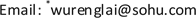1. 引言

2. 模型和研究方法

H = − γ ∑ l , l ′ ( d l + d l ′ + h . c . ) + ∑ l e V ex ( l ′ , ω ) d l + d l     + ∑ l ( U δ 〈 n l ( ω ) 〉 + V ∑ l ′ δ 〈 n l ' ( ω ) 〉 ) n l (1)

ψ m ( l ) = 8 L x L y L z sin ( m x π L x l x ) sin ( m y π L y l y ) sin ( m z π L z l z ) (2)

E m = − 2 γ cos ( m x π L x ) − 2 γ cos ( m y π L y ) − 2 γ cos ( m z π L z ) (3)

(2)和(3)式中， L x = N x + 1 ， ， ， m x = 1 , 2 , 3 , ⋯ , N x ， m y = 1 , 2 , 3 , ⋯ , N y ， m z = 1 , 2 , 3 , ⋯ , N z 。基于标准的线性响应理论，电荷数对外加电势的响应为

δ 〈 n l ( ω ) 〉 = ∑ l ′ Π ( l , l ′ , ω ) [ e V ex ( l ′ , ω ) + U δ 〈 n l ′ ( ω ) 〉 + V ∑ l ″ 〈 δ n l ″ ( ω ) 〉 ] (4)

Π ( l , l ′ , ω ) = 2 ∑ m n f ( E m ) − f ( E n ) E m − E n − ℏ ω − i η ψ m * ( l ) ψ n ( l ) ψ n * ( l ′ ) ψ m ( l ′ ) (5)

δ 〈 n l ( ω ) 〉 − ∑ l ′ , l ″ Π ( l , l ′ , ω ) v l ′ l ″ δ 〈 n l ″ ( ω ) 〉 = ∑ l ′ Π ( l , l ′ , ω ) e V ex ( l ′ , ω ) (6)

v l ″ l ′ = { U , | l ′ − l ″ | = 0 V , | l ′ − l ″ | = 1 0 , else (7)

Q ( l , ω ) − ∑ l ′ Π ( l , l ′ , ω ) v l ′ l ″ Q ( l ″ , ω ) = e 2 ∑ l ′ Π ( l , l ′ , ω ) V ex ( l ′ , ω ) (8)

(8)式为体系电荷量对外加电势的响应方程。整理(8)式可得

∑ l ′ [ δ l l ' − ∑ l ″ Π ( l , l ″ , ω ) v l ″ l ′ ] Q ( l ′ , ω ) = e 2 ∑ l ′ Π ( l , l ′ , ω ) V ex ( l ′ , ω ) (9)

P ( ω ) = ∑ l l ⋅ Q ( l , ω ) (10)

3. 计算结果

4. 结论